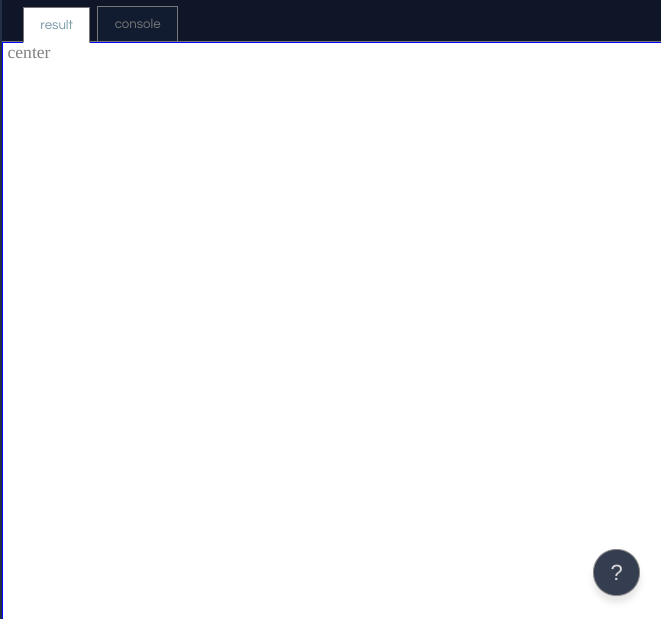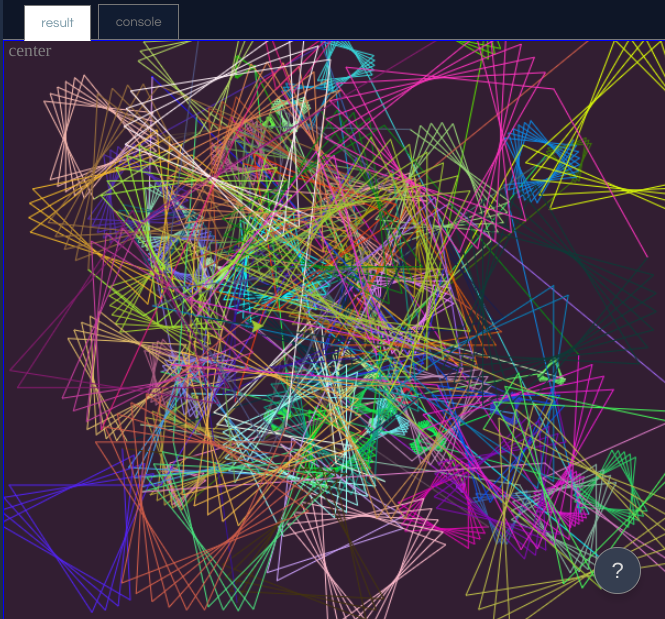RANDOM ART GENERATOR

This tutorial is meant for beginners who are getting started with Python Turtle. This will make a simple program that will make a different piece of art every time you run it. This is a really fun tutorial and I am sure you will enjoy it

### What is Turtle? and Making a New Repl

Turtle is a module in python that lets you use visual graphics. As the name suggests, Turtle draws slow, even on maximum speed. However, this adds to our program since you get to see it draw! Turtle helps you visualize programming and it is great for beginners! Without further ado, let's get on with this! On the top right corner of your screen, you should see a button called New Repl. Click that and click Python with Turtle.

### Getting Started

First, we have to import the modules needed for our code. If we were to make these modules by ourselves, it would take years and we would be reinventing something already there. So, type in
`import random`
`import turtle`
Now that we have that down, we have to create our turtle objects and the background. Think of the background like a paper, and think of the turtle like a pen. Now, whenever we move it, it leaves a trail on the paper unless we lift it, using the command `penup`, which we will get to later. Now type in
`c=turtle.Screen()`
and
`t=turtle.Turtle()`
These lines of code just generated our "paper" and our "pen". Feel free to run it. You should see something like this:Those lines of code above are going to be making our art. Also, what this just did was it created a variable, so we could mention it in the code, and it will read what it is equal to instead of just plain old c or t.
Now, let's set the speed to 1000 so that the turtle moves fast, and let's change the screen color to a nice dark magenta.
`t.speed(1000)`
`c.bgcolor(50, 30, 50)`
Now let's start loopin'! We need to add in a for loop, or a type of loop that repeats until a number that you specify. We also need to add a multicolored turtle, so we need to define random values that we can put in later. So, do

``````for i in range(1000):
r = random.randint(0,255)
g = random.randint(0,255)
b = random.randint(0,255)
t.color(r,g,b)``````

Now, we need to make our turtle move around randomly. So we need to define more variables for the x and y coordinates.

``````  x = random.randint(-200,200)
y = random.randint(-200,200)
t.goto(x,y)``````

Now, the pen will go to a random location. Now, we need to draw in the shapes. So, right now if you run the program, it will make a bunch of lines all over the place. You could leave it right there, if you wish, but I want to make the pen stop at certain places and make a shape. For this, I want a star shape. So, let's write a program that helps us make a star! We will need a side length variable, and a for loop to make the star. We need to make the side length variable always increase so that we get a nice variety of stars. So, write

``````  sideLength = i*2
for x in range(random.randint(5,20)):
t.forward(sideLength)
t.left(123)``````

In this case, the i refers to the for loop that we made earlier, and it counts the iterations. That way, it gradually increases. We multiplied it by two because the stars were too small. The number of times to for loop runs, which is being chosen randomly, is how many sides there will be in the star. During the loop, the turtle moves as far as the `sideLength` as displayed above, and then turns left by 123 degrees, making the star. Feel free to play with the degree amount, but I liked 123 the most. Now, check to make sure that every piece of code I mentioned in this tutorial below the for loop is indented and under the for loop. Whenever you indent something and put it under a loop with a colon at the end, that is the part that you loop, so make sure that your code is correctly indented. Now, we need to do the last line of code, which lifts the pen up and makes the pen move elsewhere without making a mark.
`t.penup`
Feel free to run the program, and look at your art. On mine, it looks like this:I have a channel called funcoding14. The link to it is above. However, I am making new videos on a channel called Tech Tips, in where I will also make 3d modeling tutorials. I hope you enjoyed this tutorial. If you viewed this tutorial, please upvote it. It means a lot.

You are viewing a single comment. View All